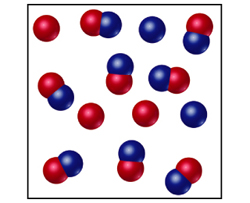# Problem: The following diagram represents an equilibrium mixture produced for a reaction of the type A + X .Is K greater or smaller than 1 if the volume is 1 L and each atom/molecule in the diagram represents 1 mol?

###### FREE Expert Solution

Recall that if K > 1, full dissociation is expected

For K < 1, partial dissociation is observed

94% (293 ratings)###### Problem Details

The following diagram represents an equilibrium mixture produced for a reaction of the type A + X .Is K greater or smaller than 1 if the volume is 1 L and each atom/molecule in the diagram represents 1 mol?

Frequently Asked Questions

What scientific concept do you need to know in order to solve this problem?

Our tutors have indicated that to solve this problem you will need to apply the Chemical Equilibrium concept. You can view video lessons to learn Chemical Equilibrium. Or if you need more Chemical Equilibrium practice, you can also practice Chemical Equilibrium practice problems.

What professor is this problem relevant for?

Based on our data, we think this problem is relevant for Professor Hurst's class at LSU.